# 5 3 Solving Quadratic Equations by Factoring WRITE

• Slides: 145. 3 Solving Quadratic Equations by Factoring WRITE QUADRATIC EQUATIONS IN INTERCEPT FORM. • SOLVE QUADRATIC EQUATIONS BY FACTORING •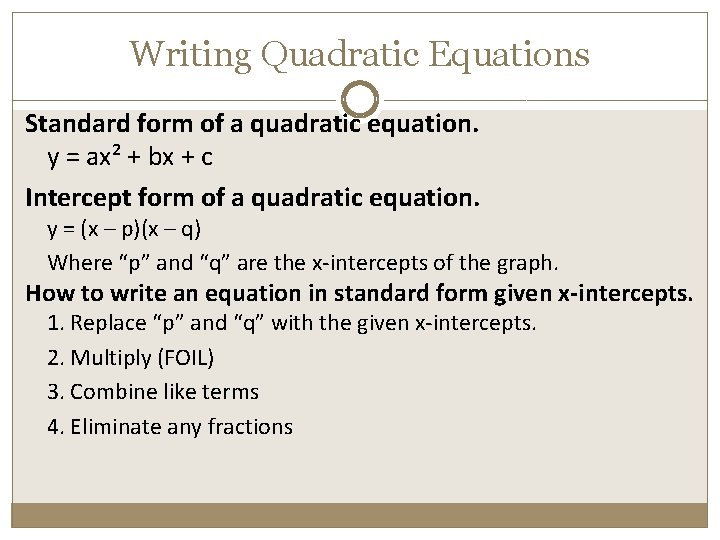Writing Quadratic Equations Standard form of a quadratic equation. y = ax² + bx + c Intercept form of a quadratic equation. y = (x – p)(x – q) Where “p” and “q” are the x-intercepts of the graph. How to write an equation in standard form given x-intercepts. 1. Replace “p” and “q” with the given x-intercepts. 2. Multiply (FOIL) 3. Combine like terms 4. Eliminate any fractions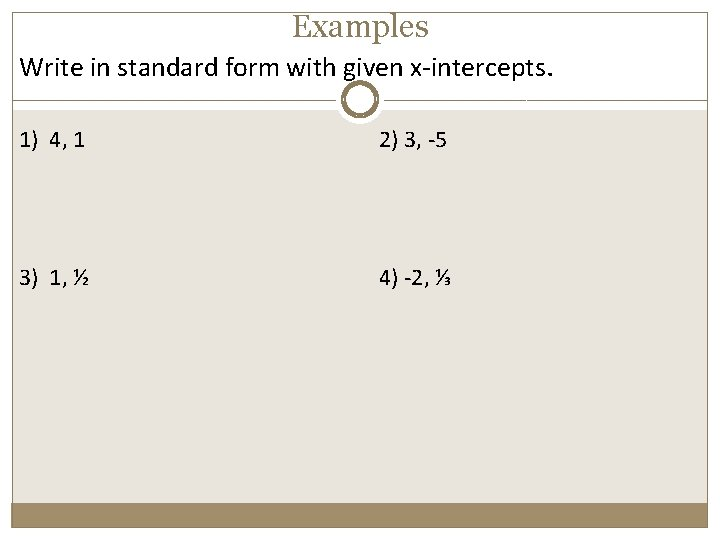Examples Write in standard form with given x-intercepts. 1) 4, 1 2) 3, -5 3) 1, ½ 4) -2, ⅓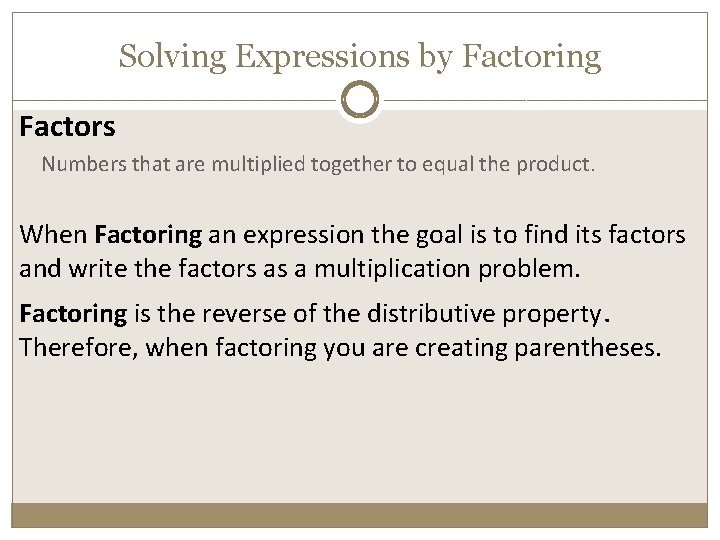Solving Expressions by Factoring Factors Numbers that are multiplied together to equal the product. When Factoring an expression the goal is to find its factors and write the factors as a multiplication problem. Factoring is the reverse of the distributive property. Therefore, when factoring you are creating parentheses.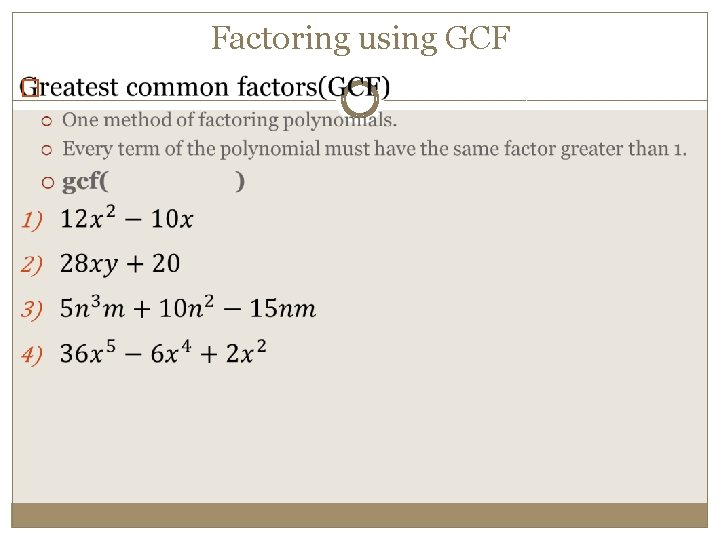Factoring using GCF �Factoring using Difference of Squares �Factoring Trinomials �� Factoring Trinomials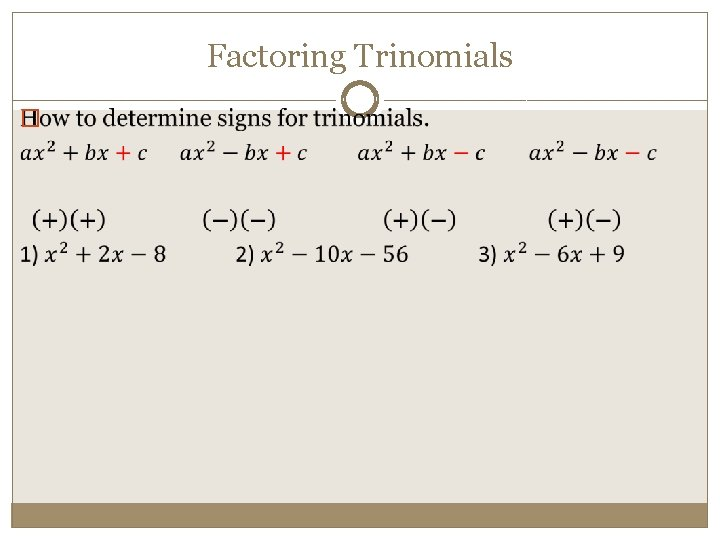Factoring Trinomials �Factoring Trinomials with lead coefficient �Factor each polynomial completely 1) y² - 81 2) x² + x – 30 3) x² + 4 x + 3 4) 6 x² - 27 x + 9 x⁵ 5) 4 xy² - 16 x 6) x² - 2 x - 3Factoring Perfect Cubes x³ - y³ = (x – y)(x² + xy + y²) x³ + y³ = (x + y)(x² - xy + y²) Factor Hint rewrite numbers as a perfect cubes then factor. 1) x³ + 27 2) y³ - 125 3) 8 x³ - y³Solving quadratic equations by factoring How to Solve Quadratic Equations 1. Graph and find the x-intercepts 2. Set equation equal to zero, factor, and use the zero product property. Zero Product Property For any real numbers “a” and “b”, if a • b = 0, then either a = 0 OR b = 0. If (x + 5)(x – 7) = 0, then x + 5 = 0 OR x – 7 = 0 If 2 x(3 x – 5) = 0, then 2 x = 0 OR 3 x – 5 = 0Solve by factoring �